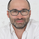# MULTIPLE TIME-FRAME STRATEGY(TREND, MOMENTUM, ENTRY)

12754 views
Hey everyone, this is one strategy that I have found profitable over time. It is a multiple time frame strategy that utilizes 3 time-frames. Highest time-frame is the trend, medium time-frame is the momentum and short time-frame is the entry point.

Long Term:
- If closed candle is above entry then we are looking for longs, otherwise we are looking for shorts

Medium Term:
- If Stoch SmoothK is above or below SmoothK and the momentum matches long term trend then we look for entries.

Short Term:
- If a moving average crossover(long)/crossunder(short) occurs then place a trade in the direction of the trend.

- Trade is closed when the Medium term SmoothK Crosses under/above SmoothD.

Best of luck!
```//@version=2
strategy("TUX MTF", overlay=true)

// MULTIPLE TIME FRAME STRATEGY
// LONG TERM --- TREND
// MED TERM --- MOMENTUM
// SHORT TERM --- ENTRY

// ENTRY POSITION TIMEFRAME
entry_position = input(title="Entry timeframe (minutes)", type=integer, defval=5, minval=1, maxval=1440)
med_term = entry_position * 4
long_term = med_term * 4

// GLOBAL VARIABLES
ma_trend = input(title="Moving Average Period (Trend)", type=integer, defval=50, minval=5, maxval=200)

// RSI
length = input(title="Stoch Length", type=integer, defval=18, minval=5, maxval=200)
OverBought = input(title="Stoch OB", type=integer, defval=80, minval=60, maxval=100)
OverSold = input(title="Stoch OS", type=integer, defval=20, minval=5, maxval=40)
smoothK = input(title="Stoch SmoothK", type=integer, defval=14, minval=1, maxval=40)
smoothD = input(title="Stoch SmoothD", type=integer, defval=14, minval=1, maxval=40)
maSm = input(title="Moving Avg SM", type=integer, defval=7, minval=5, maxval=50)
maMed = input(title="Moving Avg MD", type=integer, defval=21, minval=13, maxval=200)

// LONG TERM TREND
long_term_trend = security(ticker, tostring(long_term), sma(close,ma_trend)) > security(ticker, tostring(long_term), close)
plot(security(ticker, tostring(long_term), sma(close,ma_trend)), title="Long Term MA", linewidth=2)
// FALSE = BEAR
// TRUE = BULL

// MED TERM MOMENTUM

k = security(ticker, tostring(med_term), sma(stoch(close, high, low, length), smoothK))
d = security(ticker, tostring(med_term), sma(k, smoothD))

os = k >= OverBought or d >= OverBought
ob = k <= OverSold or d <= OverSold

// SHORT TERM MA X OVER
bull_entry = long_term_trend == false and os == false and ob == false and k > d and security(ticker, tostring(entry_position), crossover(sma(close, maSm), sma(close, maMed)))
bear_entry = long_term_trend == true and os == false and ob == false and k < d and security(ticker, tostring(entry_position), crossunder(sma(close, maSm), sma(close, maMed)))

bull_exit = crossunder(k,d)
bear_exit = crossover(k,d)

if (bull_entry)
strategy.entry("Long", strategy.long, 10000)

if (bear_entry)
strategy.entry("Short", strategy.short, 10000)

strategy.close("Long", when = bull_exit == true)
strategy.close("Short", when = bear_exit == true)

```

## KommentareThis strategy rely on future data (repaints) and can not be used in real life. Please vote up for fixing this issue: https://getsatisfaction.com/tradingview/topics/strategies-and-indicators-are-repainting
Antworten